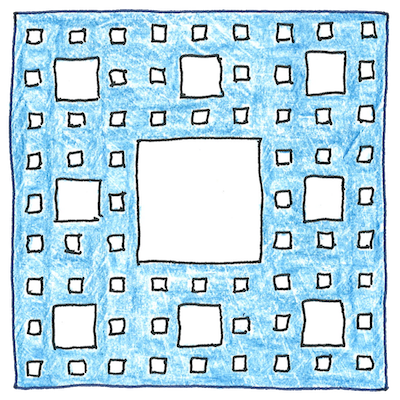# 1975

## The book of science

Tom Sharp

 Benoit Mandelbrot mathematics

## Fractal

• A fractal is
• a geometric figure
• that is similar to itself
• at all scales.
• Bending a line
• into a fractal shape
• in two dimensions
• approaches a surface.
• Breaking a surface
• into a fractal shape
• in three dimensions
• approaches a solid.
• Benoit Mandelbrot
• coined the term fractal
• from the Latin word
• meaning “fractured.”

## Sierpinski carpet

• Divide a square
• into nine subsquares,
• remove the center square,
• and repeat indefinitely.
• The result is a perimeter
• containing zero area.
• Its interior, eventually,
• is totally empty.

## Koch snowflake

• Replace the middle third
• of each side
• of an equilateral triangle
• with two sides
• of an equilateral triangle
• pointing outward.
• Repeating indefinitely
• results in a finite area
• bounded by an infinitely long line.

## Mandelbrot set

• The Mandelbrot set contains
• the complex numbers that when added
• to z2 continue to give finite values
• as z goes from zero to infinity,
• so they can be mapped
• to a two-dimensional colored image.
• Mandelbrot did not invent the Mandelbrot set.
• who also invented the Douady rabbit,
• which is similar to the Mandelbrot set
• but has a pair of floppy ears instead of buds,
• and each ear has a pair of ears, ad infinitum.

## Natural fractals

• A human brain,
• balls of crumpled paper,
• the inner surface of human lungs,
• distribution of galaxy clusters,
• the coast of Ireland,
• approximate fractals
• of different fuzzinesses,
• which is why fractals are used
• in computer graphic systems
• to approximate natural objects.

Benoit Mandelbrot created the first theory of roughness and proposed fractals as models of roughness in the real world.

The result of writing, producing draft after draft, also resembles a fractal; however, its depth is limited by the writer’s time and patience.